2016-07-03 10:22:12 u012363005 阅读数 5339
• ###### 全新 PowerDesigner 16.6 数据库设计与建模（精讲版）

PowerDesigner数据库设计与建模，本课程讲述了如何使用PowerDesigner进行数据库分析与建模。包括企业架构及业务流程分析，实体关系模型设计，面向对象和数据库建模的集成等功能模块进行项目需求分析、结构规划、生成框架代码，以及如何从现有系统逆向转工程代码，生成所需系统模型的全过程。软件设计师专题课程的第一篇<<软件设计与建模>>请参看https://edu.csdn.net/course/detail/24752。本课程作者联络QQ:494657271

11399 人正在学习 去看看 言文
三天的比赛结束了。今年的题比较变态，我已经被虐到无力吐槽了。。不过真是不到最后逼自己一把成不了事。最后一天突破了好多的困难。在最后的一小时里写完了论文，完成了全部的任务。

一开始报选修课的时候选matlab的原因是想学一下matlab的图像处理。然后课上一直讲的是基础。。没想到接触到图像处理是在数学建模的比赛中。。。第二天和第三天啥也没干，就在死抠图像处理程序。最终在第三天晚上完美解决。从第一天搜集的大量文献中总结筛选，最终决定了“原始照片 → 双边滤波处理（去噪）→ 切割分块取均值后重组图像 → Canny算子边缘检测 → 对比”的最佳线路。1 2 3 4 5 6 7 8 9 10 11 12 13 14 15 16 17 18 19 20 21 22 23 24 25 26 27 28 29 30 31 32 33 34 35 36 37 38 39 40 41 42 43 44 45 46 %%%%%%%%图5.6程序%%%%%%%%% clear all; close all; clc; img=imread('附件3 距2400m处的数字高程图.tif'); % img=mat2gray(img);%2值化    %%%%%%%%%%第一个图：显示原始图像%%%%%%%%%%%% subplot(121); imshow(img); title('原始图像','position',[1150,2600]); %%%%%%%%%%%%%%%%%%%%%%%%%%%%%%%%%%    %%%%%%%%%%%%%%%%%%%%%双边滤波%%%%%%%%%%%%%%%%%%%%%%% r=10;        %模板半径 [m n]=size(img); imgn=zeros(m+2*r+1,n+2*r+1); imgn(r+1:m+r,r+1:n+r)=img; imgn(1:r,r+1:n+r)=img(1:r,1:n);                 %扩展上边界 imgn(1:m+r,n+r+1:n+2*r+1)=imgn(1:m+r,n:n+r);    %扩展右边界 imgn(m+r+1:m+2*r+1,r+1:n+2*r+1)=imgn(m:m+r,r+1:n+2*r+1);    %扩展下边界 imgn(1:m+2*r+1,1:r)=imgn(1:m+2*r+1,r+1:2*r);       %扩展左边界 sigma_d=2; sigma_r=0.1; [x,y] = meshgrid(-r:r,-r:r); w1=exp(-(x.^2+y.^2)/(2*sigma_d^2));     %以距离作为自变量高斯滤波器 h=waitbar(0,'图像双边滤波中...'); for i=r+1:m+r     for j=r+1:n+r                 w2=exp(-(imgn(i-r:i+r,j-r:j+r)-imgn(i,j)).^2/(2*sigma_r^2)); %以周围和当前像素灰度差值作为自变量的高斯滤波器         w=w1.*w2;         s=imgn(i-r:i+r,j-r:j+r).*w;         imgn(i,j)=sum(sum(s))/sum(sum(w));     end     waitbar(i/m); end close(h) %%%%%%%%%%%%%%%%%%%%%%%%%%%%%%%%%%%%%%%%%%%    %%%%%%%%%%%%%%第二个图：滤波后图像%%%%%%%%%%%%%%%%% % figure; img1=mat2gray(imgn(r+1:m+r,r+1:n+r)); subplot(122); imshow(img1); title('双边滤波之后的图像','position',[1160,2600]); %%%%%%%%%%%%%%%%

Canny算子边缘检测1 2 3 4 5 6 7 8 9 10 11 12 13 14 15 16 17 18 19 20 21 22 23 24 25 26 27 28 29 30 31 32 33 34 35 36 37 38 39 40 41 42 43 44 45 46 47 48 49 50 51 52 %%%%%%%%%%%%%%%%%图5.7程序%%%%%%%%%%%%%%%%%%%%%%%%%%% clear all; close all; clc; img=imread('附件3 距2400m处的数字高程图.tif'); % img=mat2gray(img);%2值化    %%%%%%%%%%%%%%%%%%%%%双边滤波%%%%%%%%%%%%%%%%%%%%%%% r=10;        %模板半径 [m n]=size(img); imgn=zeros(m+2*r+1,n+2*r+1); imgn(r+1:m+r,r+1:n+r)=img; imgn(1:r,r+1:n+r)=img(1:r,1:n);                 %扩展上边界 imgn(1:m+r,n+r+1:n+2*r+1)=imgn(1:m+r,n:n+r);    %扩展右边界 imgn(m+r+1:m+2*r+1,r+1:n+2*r+1)=imgn(m:m+r,r+1:n+2*r+1);    %扩展下边界 imgn(1:m+2*r+1,1:r)=imgn(1:m+2*r+1,r+1:2*r);       %扩展左边界 sigma_d=2; sigma_r=0.1; [x,y] = meshgrid(-r:r,-r:r); w1=exp(-(x.^2+y.^2)/(2*sigma_d^2));     %以距离作为自变量高斯滤波器 h=waitbar(0,'图像双边滤波中...'); for i=r+1:m+r     for j=r+1:n+r                 w2=exp(-(imgn(i-r:i+r,j-r:j+r)-imgn(i,j)).^2/(2*sigma_r^2)); %以周围和当前像素灰度差值作为自变量的高斯滤波器         w=w1.*w2;         s=imgn(i-r:i+r,j-r:j+r).*w;         imgn(i,j)=sum(sum(s))/sum(sum(w));     end     waitbar(i/m); end close(h) %%%%%%%%%%%%%%%%%%%%%%%%%%%%%%%%%%%%%%%%%%%    %%%%%%%%%%%%%%第二个图：滤波后图像%%%%%%%%%%%%%%%%% % figure; img1=mat2gray(imgn(r+1:m+r,r+1:n+r)); subplot(121); imshow(img1); title('未Canny处理前的图像','position',[1200,2600]) %%%%%%%%%%%%%%%%%%%%%%%%%%%%%%%%%%%%%%%%%%%%%%%%       %%第四个图：第二个图滤波后图像的canny边缘检测处理结果%% % canny边缘检测 h2=waitbar(0,'绘制双边滤波之后的canny图...'); img4 = edge(img1,'canny');  % 调用canny函数 waitbar(3); subplot(122) imshow(img4);  % 显示分割后的图像，即梯度图像 title('Canny处理后图像','position',[1200,2600]) close(h2); %%%%%%%%%%%%%%%%%%%%%%%%%%%%%%%%%%%%%%%%%%%%%%%%%%%1 2 3 4 5 6 7 8 9 10 11 12 13 14 15 16 17 18 19 20 21 22 23 24 25 26 27 28 29 30 31 32 33 34 35 36 37 38 39 40 41 42 43 44 45 46 47 48 49 50 51 52 53 54 55 56 57 58 59 60 61 62 63 64 65 66 67 68 69 70 71 72 73 74 %%%%%%%%%%%%%%%%图5.8程序%%%%%%%%%%%%%%%%%%%%%%%%%%%% clear all; close all; clc; img=imread('附件3 距2400m处的数字高程图.tif');       %%%%%%%%%%%%%%%%%%%%%双边滤波%%%%%%%%%%%%%%%%%%%%%%% r=10;        %模板半径 [m n]=size(img); imgn=zeros(m+2*r+1,n+2*r+1); imgn(r+1:m+r,r+1:n+r)=img; imgn(1:r,r+1:n+r)=img(1:r,1:n);                 %扩展上边界 imgn(1:m+r,n+r+1:n+2*r+1)=imgn(1:m+r,n:n+r);    %扩展右边界 imgn(m+r+1:m+2*r+1,r+1:n+2*r+1)=imgn(m:m+r,r+1:n+2*r+1);    %扩展下边界 imgn(1:m+2*r+1,1:r)=imgn(1:m+2*r+1,r+1:2*r);       %扩展左边界 sigma_d=2; sigma_r=0.1; [x,y] = meshgrid(-r:r,-r:r); w1=exp(-(x.^2+y.^2)/(2*sigma_d^2));     %以距离作为自变量高斯滤波器 h=waitbar(0,'图像双边滤波中...'); for i=r+1:m+r     for j=r+1:n+r                 w2=exp(-(imgn(i-r:i+r,j-r:j+r)-imgn(i,j)).^2/(2*sigma_r^2)); %以周围和当前像素灰度差值作为自变量的高斯滤波器         w=w1.*w2;         s=imgn(i-r:i+r,j-r:j+r).*w;         imgn(i,j)=sum(sum(s))/sum(sum(w));     end     waitbar(i/m); end close(h)    %%%%%%%%%%%%%%第二个图：滤波后图像%%%%%%%%%%%%%%%%% img1=mat2gray(imgn(r+1:m+r,r+1:n+r));       %%%%%%%%%%滤波后图像高程值分块单位化并求均值%%%%%%%%% a=zeros(575); b=zeros(575); c=zeros(575); d=zeros(575); H=waitbar(0,'滤波后图像高程值分块单位化并求均值...');%粗壁障，采用4*4计算单位。 for i=1:1:575;     for j=1:1:575;         a(j,i)=img1(4*j-1,4*i-1);         b(j,i)=img1(4*j-1,4*i);         c(j,i)=img1(4*j,4*i-1);         d(j,i)=img1(4*j,4*i);         a1=a(j,i);b1=b(j,i);c1=c(j,i);d1=d(j,i);         img2(j,i)=(a1+b1+c1+d1)/16;% 取平均数之后     end     waitbar(i/575); end close(H); %%%%%%%%%%%%%%%%%%%%%%%%%%%%%%%%%%%%%%%%%%%%%%%%%       %%%%第三个图：滤波后图像高程值分块单位化后均值排列图%%%%%% % figure(1); % imagesc(img1); % figure(2); subplot(121) imshow(img2); title('未Canny处理前的图像','position',[287,630])          %%%%%%第五个图：第三个图的canny边缘检测处理结果%%%%%%% % canny边缘检测 img5 = edge(img2,'canny');  % 调用canny函数 subplot(122); imshow(img5);  % 显示分割后的图像，即梯度图像 title('Canny处理后图像','position',[287,635]) %%%%%%%%%%%%%%%%%%%%%%%%%%%%%%%%%%%%%%%%%%%%%%%%%%%

2019-09-28 10:35:35 xiaotang_sama 阅读数 35
• ###### 全新 PowerDesigner 16.6 数据库设计与建模（精讲版）

PowerDesigner数据库设计与建模，本课程讲述了如何使用PowerDesigner进行数据库分析与建模。包括企业架构及业务流程分析，实体关系模型设计，面向对象和数据库建模的集成等功能模块进行项目需求分析、结构规划、生成框架代码，以及如何从现有系统逆向转工程代码，生成所需系统模型的全过程。软件设计师专题课程的第一篇<<软件设计与建模>>请参看https://edu.csdn.net/course/detail/24752。本课程作者联络QQ:494657271

11399 人正在学习 去看看 言文

# 基于 MATLAB 的数字图像处理模型

### 1 摘要

• 对于问题一，将人脸部分的像素点三维矩阵分为若干个小矩阵，对每个小矩 阵内的所有像素点赋予相同的 RGB值，即可得到马赛克效果。为了对比效果，分 别使用了均值法，左上角值法，随机值法进行处理，又对小矩阵大小分别设置为3×3、4×4、5×5。最终得到小矩阵大小为 4×4 时的均值法得到的马赛克效果 最好，最能够符合题目要求。

• 对于问题二，根据计算机对于灰度处理的基本步骤，主要工作为将像素点的 R、G、B值置为一相等值，对于原图，分别使用加权平均法、平均值法、最大值 法求出了对应灰度值，最终确定加权平均法所得灰度图效果最为适宜。

• 对于问题三，为简化提取轮廓线过程，首先将 RGB 图像转化为灰度图。然后 使用 sobel 算子以及 prewitt算子对图像进行卷积，得到的梯度值矩阵经过归一 化处理以及灰度化处理，得到基本轮廓图。为使图片轮廓效果更好，通过灰度转置以及直方图均衡化操作，得到最终效果图。

• 对于问题四，要放大图片，即要对各像素点的 RGB 值进行分层插值处理，对
图片分别采取双线插值法和最邻近插值法进行放大操作，对比得到双线插值法放 大的图像更加清晰。

• 对于问题五，通过对于装饰图的二值化处理，得到装饰图的前景与后景的分 离效果。对于图像的合成，采用了像素点 RGB
值替换的做法，将装饰图中呈现为 黑色的部分定位坐标，再将原彩色图的对应像素替换人物图片中的指定像素点。
而在定位替换点的时候，通过手动测量和识别人脸自动定位两种方法进行定位， 得出两个符合题意的合成图片。

### 2 具体过程

#### （1）问题一：马赛克的处理

• 首先，我们需要知道一般图像处理中，对图像进行读取以及后续处理的原理，这也是整个建模过程的基础：
对于图像来说，图像是成千上万个像素点构成，每一个像素点，具有三个维度的信息，我们称之为RGB色彩值，RGB的意思就是三原色，红色red，绿色green，蓝色blue，这三原色可以构成我们平常所看到的颜色。它们的取值是（0，255）
• 现在我们可以了解马赛克的原理：马赛克的原理有许多种，本文参考的是文献【1】提供的均值处理方法，简单来说，就是将需要处理的图像区域分成若干个小像素块，假设任意的一个小像素区域为A，然后对于A来说，我们对这个区域的像素RGB色彩值取均值，然后重新赋值此区域。便可以得到一个“中和”过的色彩值。（这部分RGB是分为三层处理，也就是说red层处理一次，green一次，blue一次）
• 在论文中，我们根据分块的不同尺寸进行了操作，效果如图：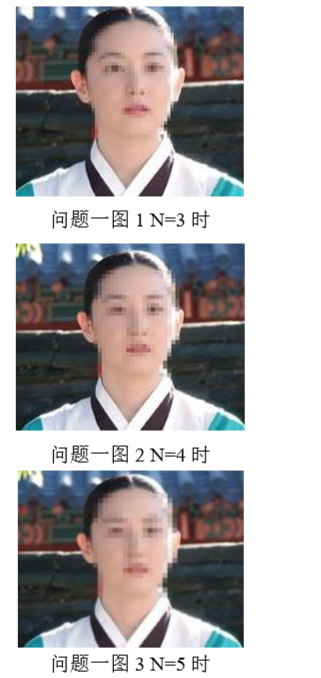可以看到，若是区域区的越大，那么图像就越模糊，因为题目要求能够模糊五官但是能够观察到人脸，我们认为像素区域取4*4的时候效果是比较好的。
• 为了马赛克效果的多样性，我们添加了不同于对新的区域的赋值方法，分别是：取左上角的色彩值为整体的方法和随机取色彩值作为新的整体色彩值，效果如下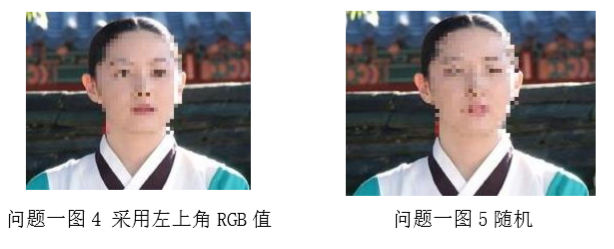效果稍微有些区别，可以看出其实有一些像我们平常使用p图软件的不同马赛克笔。

#### （2）问题二：彩图转化为灰度图

• 首先，在做题之前，我们应该需要搞清楚题目中的概念，灰度图是什么？
• 我们可以认为，灰度图是彩图在灰度值之间的一个映射，常用的转换方法有以下几种：假如原来某点的颜色为RGB(R，G，B)，那么，我们可以通过下面几种方法，将其转换为灰度：
1.浮点算法：Gray=R0.3+G0.59+B0.11
2.整数方法：Gray=(R
30+G59+B11)/100
3.移位方法：Gray =(R76+G151+B*28)>>8;
4.平均值法：Gray=（R+G+B）/3;
5.仅取绿色：Gray=G；

通过上述任一种方法求得Gray后，将原来的RGB(R,G,B)中的R,G,B统一用Gray替换，形成新的颜色RGB(Gray,Gray,Gray)，用它替换原来的RGB(R,G,B)就是灰度图了
• 我们可以观察到，灰度图的三维都是相同的
• 在建立模型时，我们采用了三种方法，分别是浮点算法（权值平均法），均值法，以及最大值法（未在上述方法中列出），处理后，通过matlab的imshow命令，效果如下：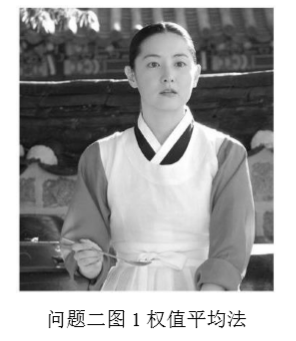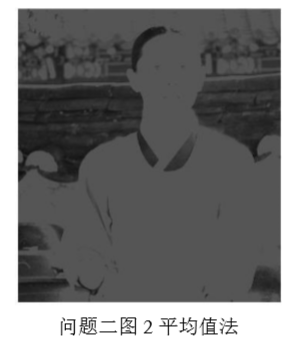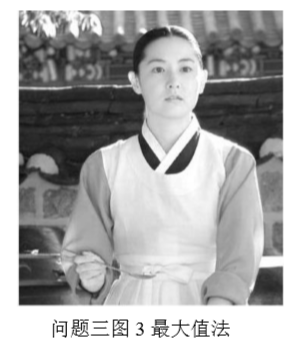### （3）问题三：轮廓提取

• 题目要求是线描图，其实转成图像处理的问题，就是对于整个图片的轮廓提取。

• 那么如何实现轮廓提取？事实上，现在已经有非常成熟的解决方法。一般来说，轮廓提取问题可以粗略的描述为找到图像中那些沿特定方向局部强度变化显著的位置（这里如果不好理解的话，我们可以认为一般在图像中，一个物体与背景，或者人与背景，颜色是有很大的不同的），提取轮廓就是保留这些边缘。在图像中我们的首要任务就是区分出相同变化梯度和不同变化梯度的区域（相同或者相近的变化梯度的地方可以认为是一个不需要分割区域）。

• 由于 RGB 图是一个三维图像，在处理的时候会比较复杂，因此先通 过第二问的求解方法来将 RGB 的空间图像转换为灰度图像

• 而对于图像中的梯度，我们有很好的工具去描述，即图像中某一横切面所对 应的偏导数构成的向量，称为梯度向量
$\nabla f=(\frac{\partial f}{\partial x},\frac{\partial f}{\partial y})\\ \ \\ ||\nabla f||=\sqrt{(\frac{\partial f}{\partial x})^{2}+(\frac{\partial f}{\partial y})^{2}}$

• 为了求得每一点的梯度值，引入 sobel 算子，利用算子对图像 进行卷积操作。（以下图片部分来自本人的比赛论文）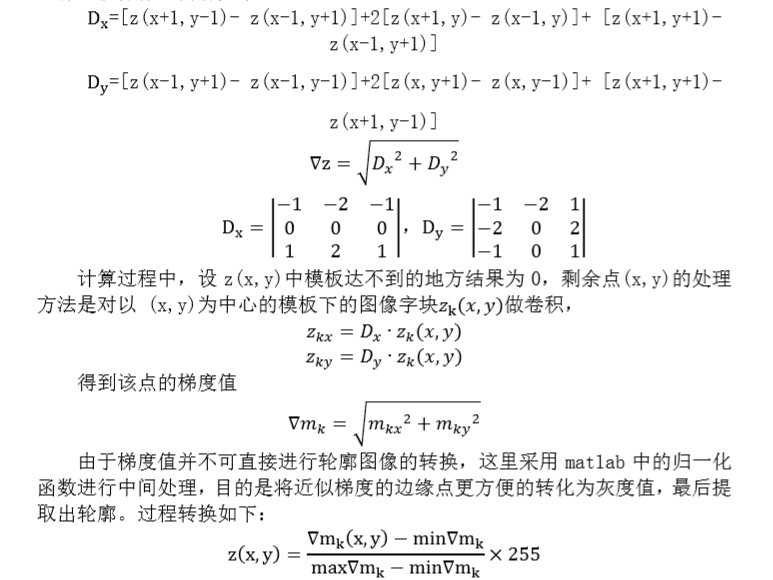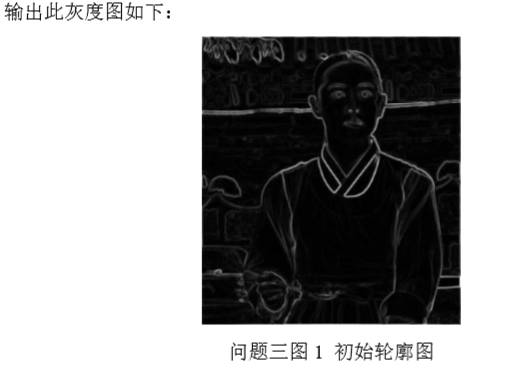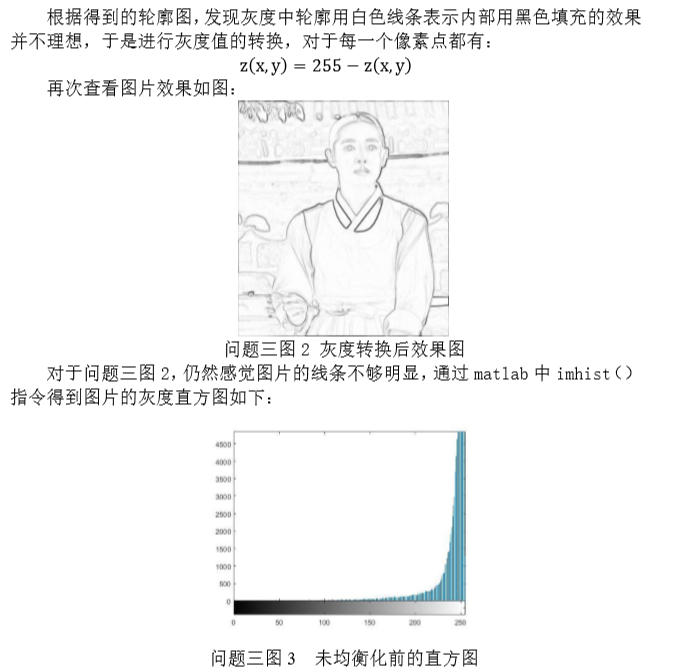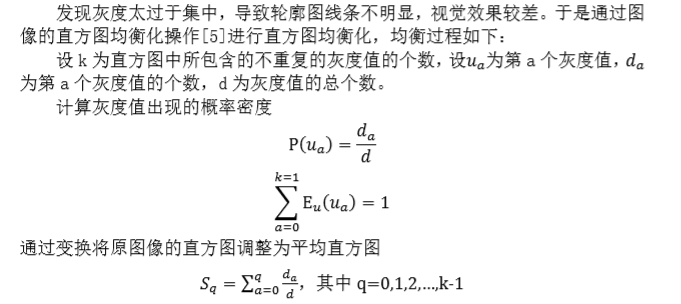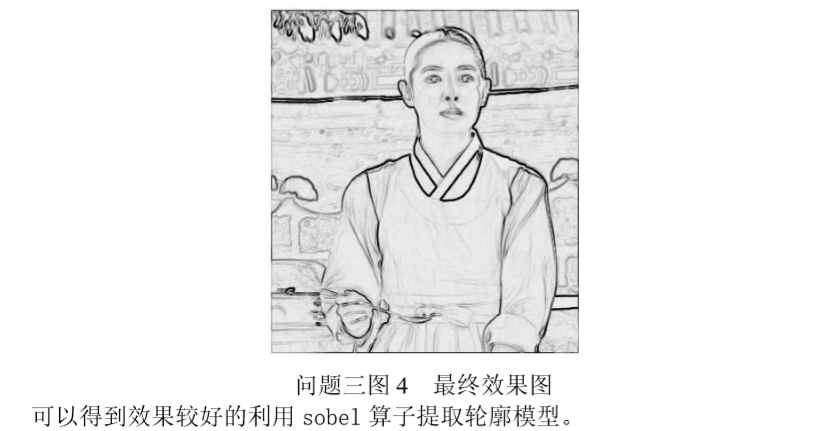• 图片的轮廓提取是个非常复杂的问题，如果想要比较好的效果，需要比较繁复的处理，博主本人也只是参考了常用的轮廓提取的方法，用了最简单的梯度向量的处理方法和后续的灰度均衡化的方法，主要的参考来源来自于文献【2】【3】，如果大家对于图像处理的细节有进一步的需求，可以再参考两本文献

#### （4）问题四：图片的放大

• 对于图像 n 倍放大，我们可以简单的认为图像的放大即为新建一个图像， 拥有原图像的 n 倍像素点数量，且每个像素点的 RGB 值都与原图中的某一对应像 素点的 RGB 产生联系(或者说：新图像的每一点，都是来自于原来的图像)。由此可以想到利用插值来得到放大图像中某一像素点所对 应的 RGB 值
• 在具体的处理中，我们使用了双线插值法以及最邻近插值法
• 事实上，最邻近插值法的效果经实验并不好，因为会造成严重的图像失真。在论文中，主要用于对比。
• 图片的缩放最核心的问题就是：放大/缩小后的图片的像素点是通过何种方法来自于原来的图片中的像素点。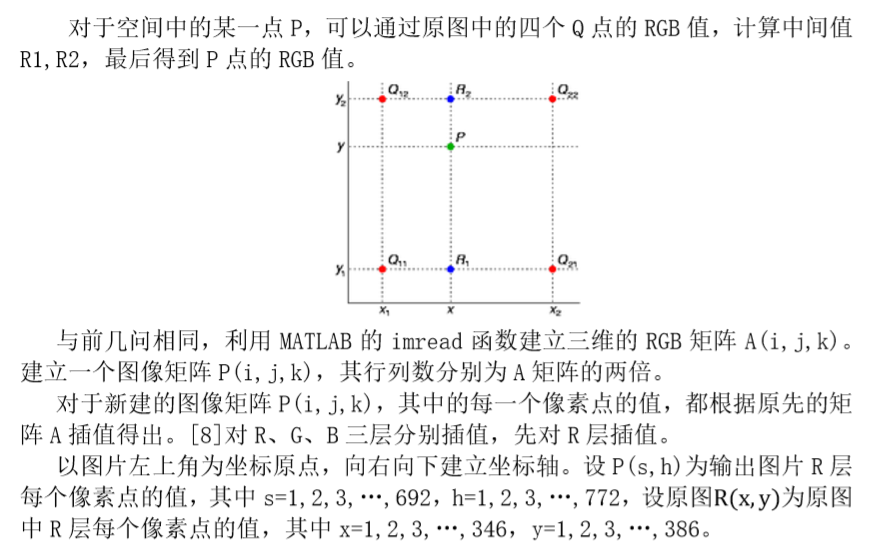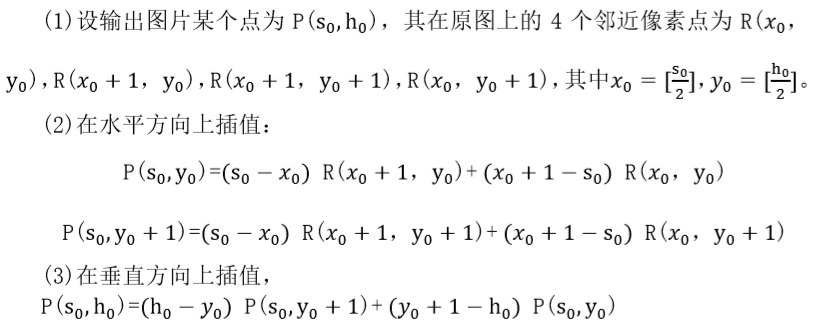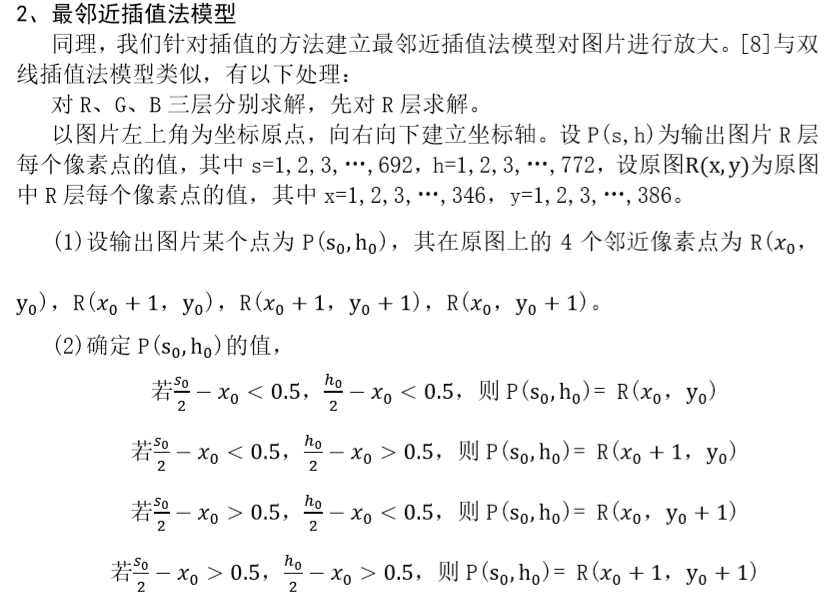#### （5）问题五：图片的分离与合成，图像定位（人脸识别）

• 在这一个问题中，实际上是分成两个部分的，第一个部分是需要将装饰物从本身的图片中分离出来，因为装饰物在一个白色背景图中，我们在合成的时候，只是需要装饰物本身，而不需要白色背景，因为我们的思路是，先将装饰物的彩图转化为二值图。然后通过二值图的0，1分布，装饰物所在的区域的位置，我们可以记录下来。
• 这个分离的问题我们可以理解为为自己戴上一副红外装置，整个世界都分成了两种，一种是0，一种是1，为0的像素点的位置，我们可以记录下来，这一点的像素值，是我们所需要的装饰物的像素值
• 第二个问题，可以简单的认为是我们需要定位在图中的人脸的位置，定位后，将装饰物放置于头顶（放置问题就是图片的合成，可以使用装饰物的像素替换头顶上方区域的像素）
##### a.图片的分离与合成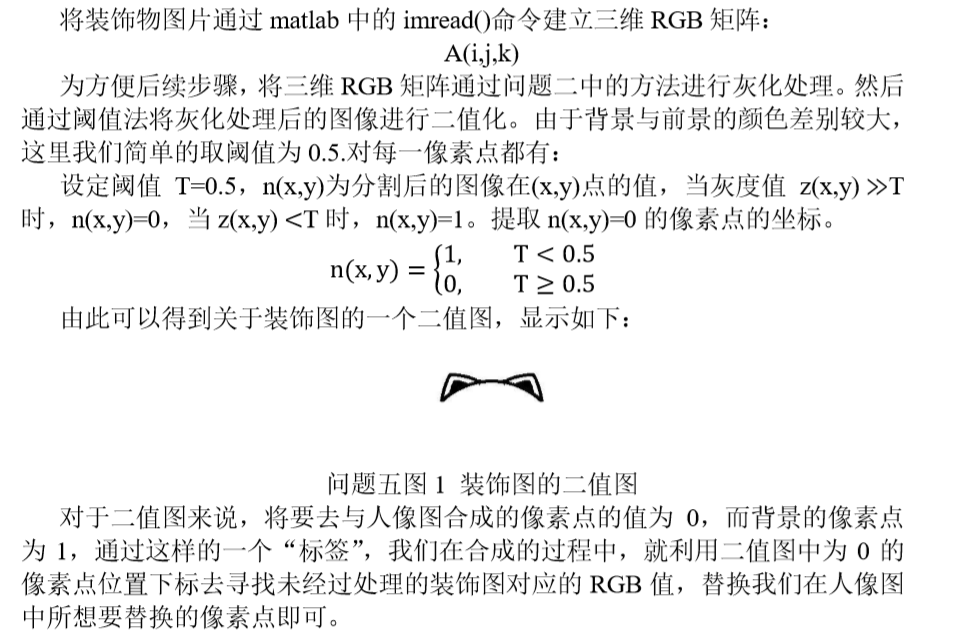##### b.定位（人脸识别）
• 主要参考的依据是YCbCr 肤色模型，可以通过肤色的亮度与周围环境的亮度来简单区分肤色与背景。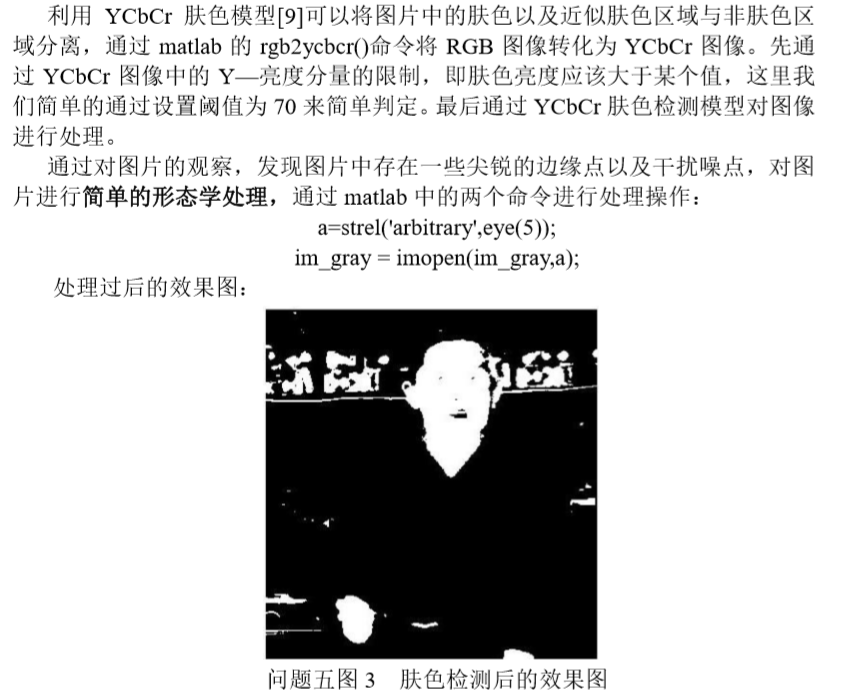• 但是，这样还是有问题，对于亮度和人脸的肤色差不多，以及手的区域，还是不能够很好的与人脸区分开来。因此我们需要加入一些别的标准来判断一个区域是否是人脸：一个白色区域到底是不是人脸，我们可以通过加入长高比（人脸是不可能达到一个非常极端的比值，以及在图片中的像素区域的大小等判别标准）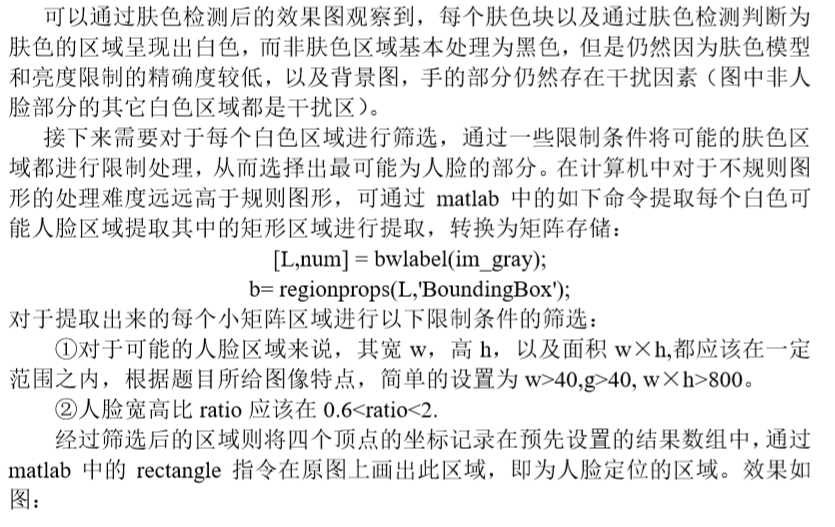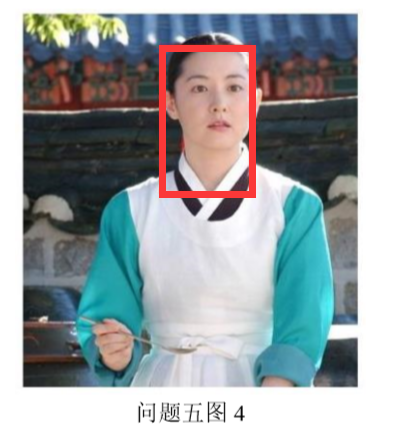接下来用像素替换即可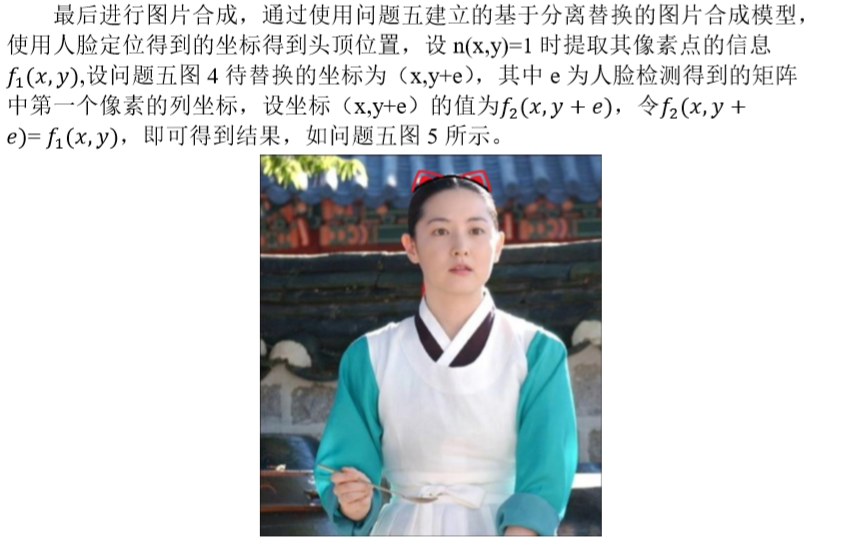### 参考文献

 马赛克原理，HTTPS://WWW.ZHIHU.COM/QUESTION/31985844，2018，5，11
 顾梅花，苏彬彬，王苗苗，王志磊, 彩色图像灰度化算法综述 , 《计算机 应用研究》, 第 36 卷第 5 期,2018  冈萨雷斯·C·拉斐尔, 伍兹·E·理查德,数字图像处理 [M],阮秋琦, 阮 宇智, 等译. 3 版. 北京: 电子工业出版社, 2011: 151-152
 [德] 伯格，[德] 伯奇 著，黄华 等 译，数字图像处理：JAVA 语言算法描 述，清华大学出版社，2011，99-103
 徐 烨 超 ， 双 线 性 插 值 (BILINEAR INTERPOLATION) 原 理 及 使 用 ， HTTPS://BLOG.CSDN.NET/HUANG1024RUI/ARTICLE/DETAILS/46545329，2018.5.12
 贺兴华,周媛媛，王继阳，周晖等 MATLAB7.X 图像处理 人民邮电出版社 46-48
 BLUE_LG ， 简 单 的 基 于 YCRCB 颜 色 空 间 的 人 脸 检 测 （ 肤 色 ）， HTTPS://WWW.CNBLOGS.COM/BLUE-LG/ARCHIVE/2011/12/07/2279879.HTML

2016-03-22 21:25:07 ding977921830 阅读数 1374
• ###### 全新 PowerDesigner 16.6 数据库设计与建模（精讲版）

PowerDesigner数据库设计与建模，本课程讲述了如何使用PowerDesigner进行数据库分析与建模。包括企业架构及业务流程分析，实体关系模型设计，面向对象和数据库建模的集成等功能模块进行项目需求分析、结构规划、生成框架代码，以及如何从现有系统逆向转工程代码，生成所需系统模型的全过程。软件设计师专题课程的第一篇<<软件设计与建模>>请参看https://edu.csdn.net/course/detail/24752。本课程作者联络QQ:494657271

11399 人正在学习 去看看 言文

真幸福滴生活在信息社会，能在网络上遇到这么多技术大牛，在目标跟踪领域的背景建模方面偶然间遇到一位博主无涯（C、.net、图像处理、算法）。既有论文和算法的详细解说，还有代码具体实现！感谢生活在信息社会，感谢无涯博主----作者：王先荣！

5. 源代码下载

1. 图像处理学习系列源代码——包括该系列文章的几乎所有代码；

1.5. 图像处理学习系列中用到的dll文件包——将这个解压缩之后放到Debug或者Release目录中；

3. 直方图

4. 非参数背景生成

5. 特征检测

6.RANSAC示例

2019-03-07 20:58:56 qq_34431303 阅读数 693
• ###### 全新 PowerDesigner 16.6 数据库设计与建模（精讲版）

PowerDesigner数据库设计与建模，本课程讲述了如何使用PowerDesigner进行数据库分析与建模。包括企业架构及业务流程分析，实体关系模型设计，面向对象和数据库建模的集成等功能模块进行项目需求分析、结构规划、生成框架代码，以及如何从现有系统逆向转工程代码，生成所需系统模型的全过程。软件设计师专题课程的第一篇<<软件设计与建模>>请参看https://edu.csdn.net/course/detail/24752。本课程作者联络QQ:494657271

11399 人正在学习 去看看 言文

 采用FPGA的机器视觉系统发展现状与趋势.原魁,肖晗,何文浩.中科院自动化所.计算机工程与应用.2010
 W. J. MacLean, “An Evaluation of the Suitability of FPGAs for Embedded Vision Systems,” 2005 IEEE Computer Society Conference on Computer Vision and Pattern Recognition (CVPR’05) - Workshops, San Diego, CA, USA, 2005, pp. 131-131.

2019-09-03 18:37:38 yl_puyu 阅读数 168
• ###### 全新 PowerDesigner 16.6 数据库设计与建模（精讲版）

PowerDesigner数据库设计与建模，本课程讲述了如何使用PowerDesigner进行数据库分析与建模。包括企业架构及业务流程分析，实体关系模型设计，面向对象和数据库建模的集成等功能模块进行项目需求分析、结构规划、生成框架代码，以及如何从现有系统逆向转工程代码，生成所需系统模型的全过程。软件设计师专题课程的第一篇<<软件设计与建模>>请参看https://edu.csdn.net/course/detail/24752。本课程作者联络QQ:494657271

11399 人正在学习 去看看 言文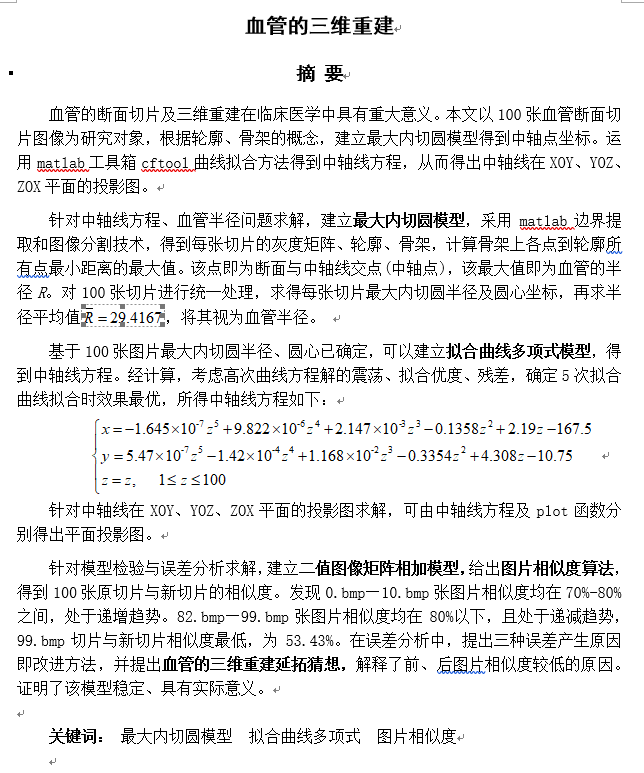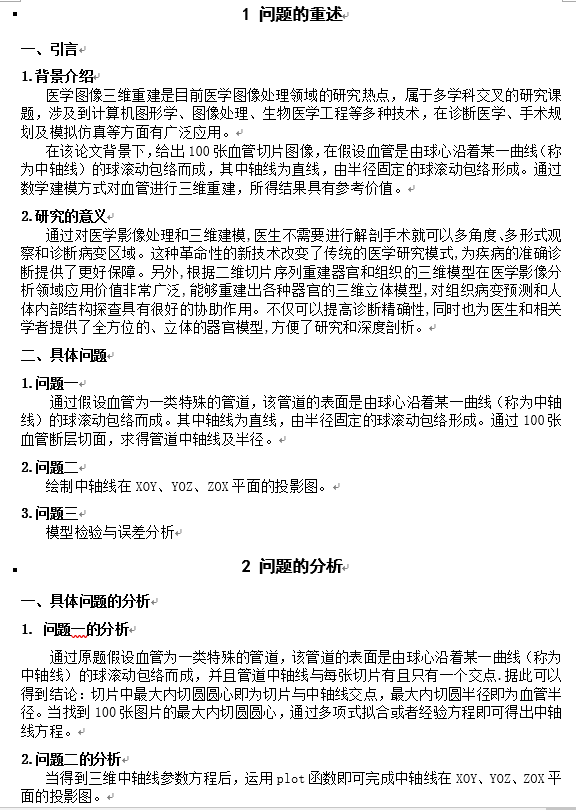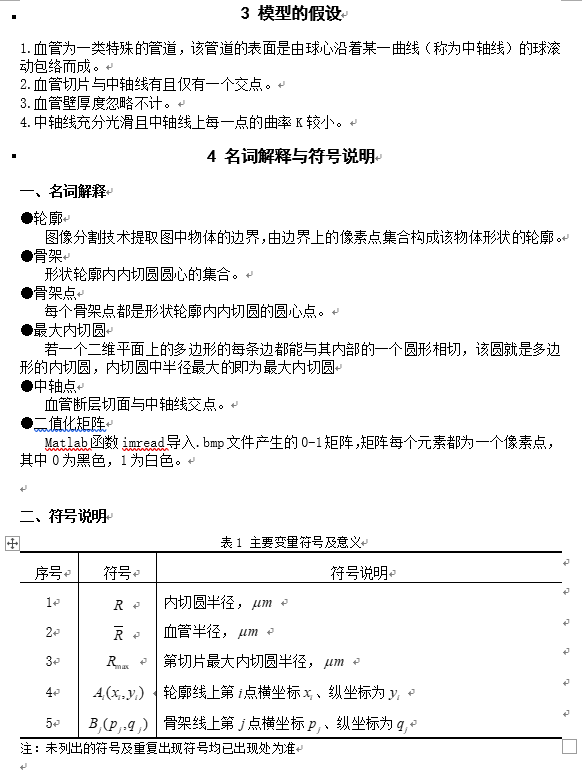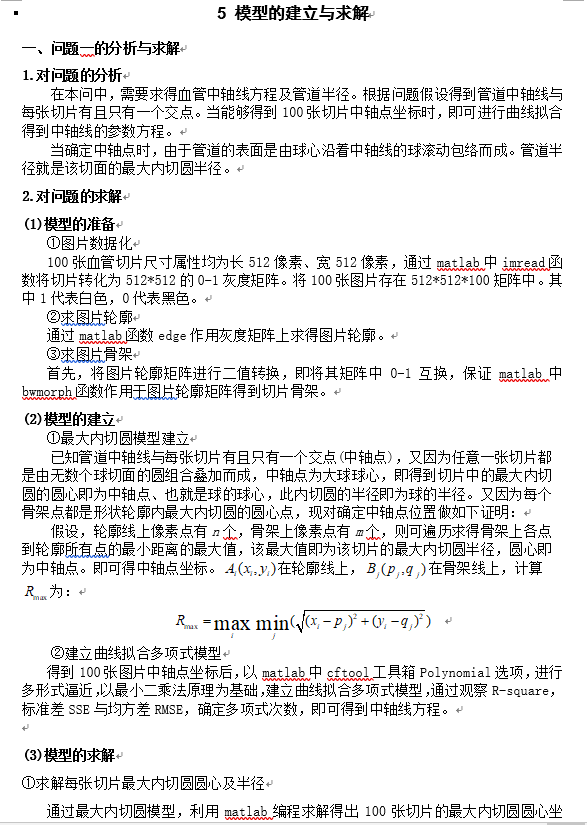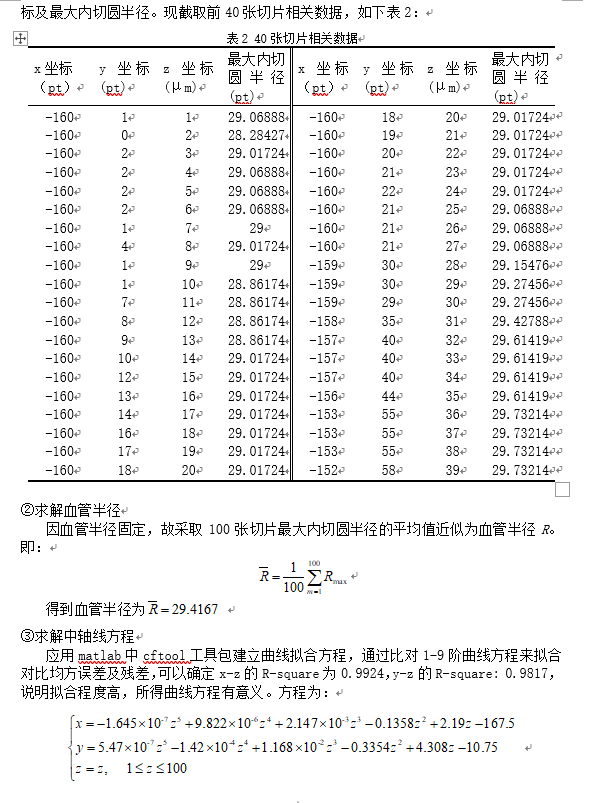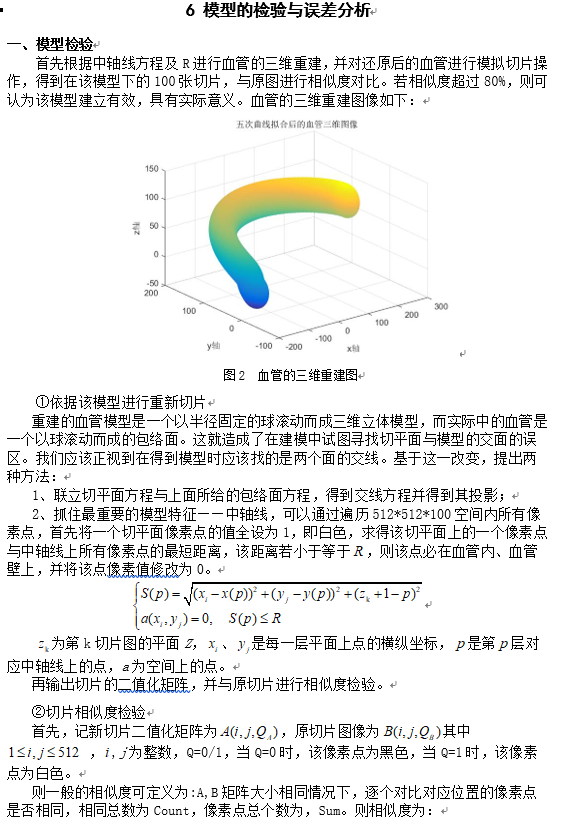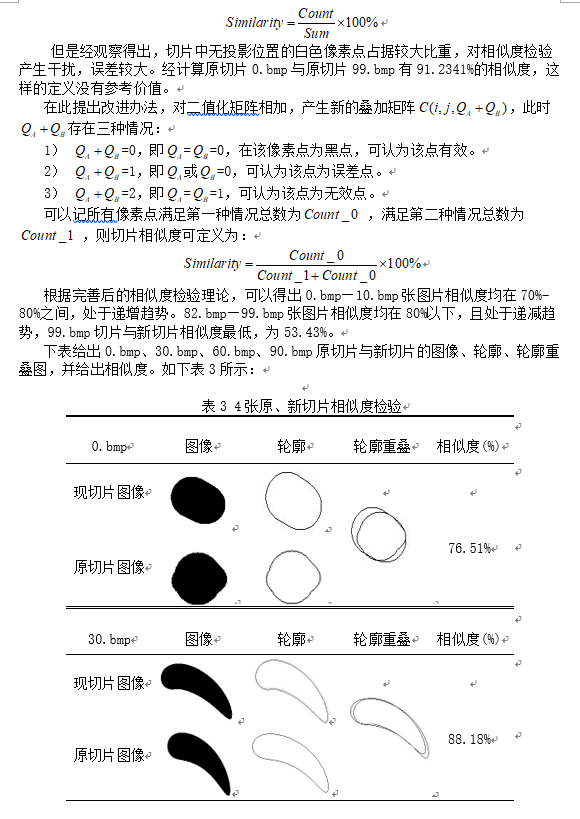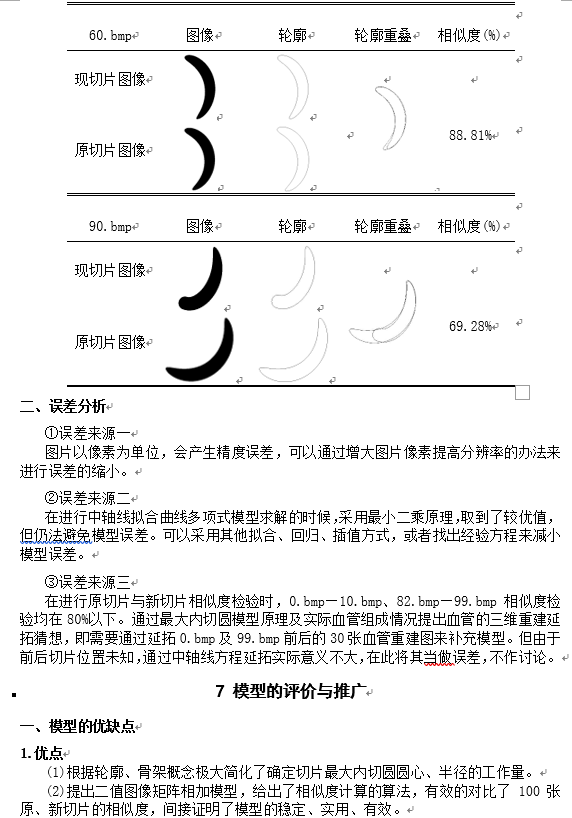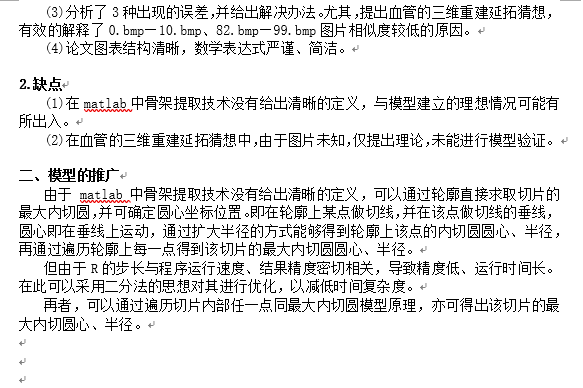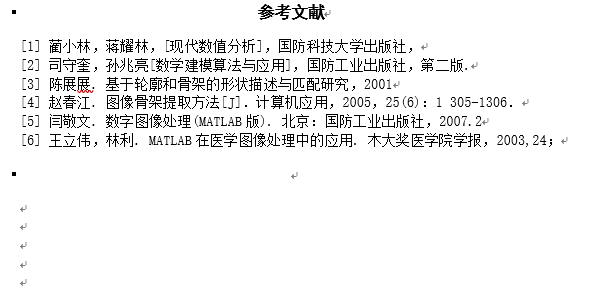clc,clear,close all
%% 读取0-99图片，并输出
for ii=0:99
imageName=strcat(num2str(ii),'.bmp'); % imageName 图片名
imdata = imread(imageName);     % imdata 灰度图像矩阵
imblack(:,:,ii+1)=imdata; % imblack 100张图片灰度矩阵以三维数组进行存储
% imdata= imshow(imageName);   %  打印图片
end
for z=1:100
for a=1:512
for j=1:512
imwhite(a,j,z)=1-imblack(a,j,z); % 1-0互换，方便提取骨架
end
end
% imshow(imwhite(:,:,z));     % 显示1-0转换后的图像
BW0(:,:,z) = edge(imwhite(:,:,z),'sobel');      % 显示图像轮廓
BW1(:,:,z) = bwmorph(imwhite(:,:,z),'skel',inf);
%BW1 将0-99图像骨架化，存入三维矩阵BW1
% BW2(:,:,z) = bwmorph(BW1(:,:,z),'spur',inf);
% BW2 将所得骨架进行消刺处理
imshow(BW1(:,:,z))        % 输出骨架图像
end
%% 求0-99图片最大内切圆及轴点、半径
Result=zeros(100,4); % Result用于存储每张图片，x、y、z坐标及最大内切圆半径R
for zz=1:100     %遍历每张图片
[x0,y0,z0]=find(BW0(:,:,zz));     %返回行，列，值（非0元素）
[a,b,c]=find(BW1(:,:,zz));     %返回行，列，值（非0元素）
max_coord=length(a);        % 返回骨架中非0元素的 个数
n=length(x0);       % 返回轮廓中非0元素的 个数
r1=zeros(max_coord,n);      % 骨架和轮廓非0元素构成 max_coord*n 的0矩阵
g=zeros(max_coord,2);       % 构造 m*2 的0矩阵
for i=1:max_coord       % 遍历每一个骨架中非0的元素
for j=1:n       % 遍历每一个轮廓中的非0元素
x1=a(i);
y1=b(i);
x2=x0(j);
y2=y0(j);
r1(i,j)=sqrt((x1-x2)^2+(y1-y2)^2); % 求骨架点到轮廓点的距离
end
[r2,coord]=min(r1(i,:)); % 求一个骨架点到所有轮廓点距离的最小值
g(i,1)=r2;
g(i,2)=coord;
end
[max_r,max_coord]=max(g(:,1));
% 求一个骨架点到所有轮廓点的距离的最小值的最大值
x=a(max_coord)-256;     % 坐标转换
y=b(max_coord)-256;     % 坐标转换

Result(zz+1,1)=x;       % x坐标存储
Result(zz+1,2)=y;       % y坐标存储
Result(zz+1,3)=zz+1;    % z坐标存储
Result(zz+1,4)=max_r;       % 半径存储
xlswrite('F:\是AAAA\matlab11\图像处理\附件A题bmp\新建.xlsx',Result);       % 路径请自行添加
end


%% 问题二 源代码（中轴线图像及投影）
% 将中轴线及三个方向上的投影显示在一个图像上
% 程序的预处理
clear %清空变量
clc % 清屏
close  % 关闭窗口
%% 函数的输入
z = 1:1:100;
x = -1.645e-07*z.^5+9.822e-06*z.^4+0.002147*z.^3-0.1358*z.^2+2.19*z-167.5;
y = 5.47e-07*z.^5-0.000142*z.^4+0.01168*z.^3-0.3354*z.^2+4.308*z-10.75;

%% 将中轴线及三个方向上的投影在一个图像上显示
subplot(2,2,1)
plot3(x,y,z)
title('图一:主轴线')
grid on
xlabel('x轴');
ylabel('y轴');
zlabel('z轴');

subplot(2,2,2)
plot(z,x)
title('图二:XOZ面投影')
grid on
xlabel('x轴');
ylabel('z轴');

subplot(2,2,3)
plot(z,y)
title('图三:YOZ面投影')
grid on
xlabel('y轴');
ylabel('z轴');

subplot(2,2,4)
plot(x,y)
title('图四:XOY面投影')
grid on
xlabel('x轴');
ylabel('y轴');


%% 第三问程序（五次曲线拟合后的血管三维图像）
%
for t=0:99
[x,y,z]=sphere(50);  % 生成球体
% 返回大小为(n+1)*(n+1)的三个矩阵中n×n球体的坐标。用surf(X,Y,Z)或mesh(X,Y,Z)绘制球体。
x=-1.645e-07.*t.^5+9.822e-06.*t.^4+0.002147.*t.^3-0.1358.*t.^2+2.19.*t-167.5+29.4167.*x;
% 最高次为五次时x与t的函数关系式
y=5.47e-07.*t.^5-0.000142.*t.^4+0.01168.*t.^3-0.3354.*t.^2+4.308.*t-10.75+29.4167.*y;
% 最高次为五次时y与t的函数关系式
z = t  + 29.4167*z;
% z与t的函数关系式
surf(x,y,z);
% 绘制球体
hold on
% 保持图像
end
%% 图像标注处理
x = xlabel('x轴');
y = ylabel('y轴');
z = zlabel('z轴');
title('五次曲线拟合后的血管三维图像')


%% 截取重建后模型的切面源代码
R=29.4924;
z=1:100;
x=-1.645e-07.*(z-1).^5+9.822e-06.*(z-1).^4+0.002147.*(z-1).^3-0.1358.*(z-1).^2+2.19.*(z-1)-167.5;
% 最高次为五次时x与t的函数关系式
y=5.47e-07.*(z-1).^5-0.000142.*(z-1).^4+0.01168.*(z-1).^3-0.3354.*(z-1).^2+4.308.*(z-1)-10.75;
% 最高次为五次时y与t的函数关系式
for m=0:99  % m代表切片层数
a=ones(512,512);  %存储截面图象灰度矩阵，全1纯白
for i=1:512 % 通过遍历i、j、p来遍历空间所以点
for j=1:512
for p=1:100
% 空间点的坐标位置遍历，血管内部的点肯定大于 （黑），外部（白）
b(p)=sqrt(((i-257)-x(p))^2+((j-257)-y(p))^2+(m+1-p)^2);
end
if min(b)<=R  % 当该点到同高度下中轴点的距离小于R时，即在血管内部
a(i,j)=0; % 染成黑色
end
end
end
imwrite(a,strcat('C:\Users\许新立\Documents\MATLAB\第一次模拟',int2str(m),'.bmp'));
% 输出切片后的图像
end


%% 最后一小问程序 源代码
%% 原图与重建模型切面的相似度百分比
clear;
clc;
%% 导入原图像及切片后图像
I3=I1+im2bw(I2); % 二值化 （矩阵相加）
count_0=0; % 记录像素为0的点（黑点）
count_1=1; % 记录像素为1的点（白点）
[h,w]=size(I3); % 读取I3的尺寸
for i=1:h
for j=1:w
if I3(i,j)==0  %逐点比较相似
count_0=count_0+1;
elseif I3(i,j)==1  %逐点比较相似
count_1=count_1+1;
end
end
end
similarity=double(count_0)/(count_0+count_1);
similarity;
%% 结果显示
%  0.bmp:similarity =
%     0.7651
% 30.bmp:similarity =
%     0.8818
% 60.bmp: similarity =
%     0.8881
% 90.bmp:similarity =
%     0.6928


%% 提取轮廓（0.bmp、30.bmp、60.bmp、90.bmp）
b= bwmorph(a,'remove');   % 提取轮廓
for i =1:512    %遍历轮廓
for j=1:512
b(i,j)=1-b(i,j);  % 0—1互换
end
end
imshow(b); % 显示去掉轮廓后的图像